## Transcendental Number

A number which is not the Root of any Polynomial equation with Integer Coefficients, meaning that it is not an Algebraic Number of any degree, is said to be transcendental. This definition guarantees that every transcendental number must also be Irrational, since a Rational Number is, by definition, an Algebraic Number of degree one.

Transcendental numbers are important in the history of mathematics because their investigation provided the first proof that Circle Squaring, one of the Geometric Problems of Antiquity which had baffled mathematicians for more than 2000 years was, in fact, insoluble. Specifically, in order for a number to be produced by a Geometric Construction using the ancient Greek rules, it must be either Rational or a very special kind of Algebraic Number known as a Euclidean Number. Because the number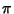is transcendental, the construction cannot be done according to the Greek rules.

Georg Cantorwas the first to prove the Existence of transcendental numbers. Liouvillesubsequently showed how to construct special cases (such as Liouville's Constant) using Liouville's Rational Approximation Theorem. In particular, he showed that any number which has a rapidly converging sequence of rational approximations must be transcendental. For many years, it was only known how to determine if special classes of numbers were transcendental. The determination of the status of more general numbers was considered an important enough unsolved problem that it was one of Hilbert's Problems.

Great progress was subsequently made by Gelfond's Theorem, which gives a general rule for determining if special cases of numbers of the form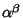are transcendental. Baker produced a further revolution by proving the transcendence of sums of numbers of the form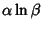for Algebraic Numbers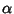and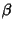.

The number e was proven to be transcendental by Hermitein 1873, and Pi () by Lindemannin 1882.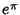is transcendental by Gelfond's Theorem since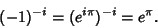The Gelfond-Schneider Constant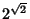is also transcendental. Other known transcendentals are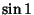where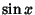is the Sine function,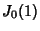where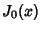is a Bessel Function of the First Kind (Hardy and Wright 1985),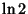,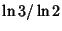, the first zero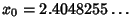of the Bessel Function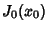(Le Lionnais 1983, p. 46),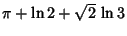(Borwein et al. 1989), the Thue-Morse Constant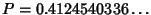(Dekking 1977, Allouche and Shallit), the Champernowne Constant 0.1234567891011..., the Thue Constant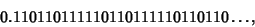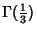(Le Lionnais 1983, p. 46),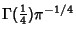(Davis 1959), and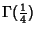(Chudnovsky, Waldschmidt), where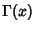is the Gamma Function. At least one of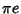and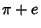(and probably both) are transcendental, but transcendence has not been proven for either number on its own.

It is not known if,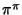,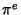,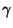(the Euler-Mascheroni Constant),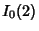, or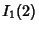(where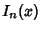is a Modified Bessel Function of the First Kind) are transcendental.

The degree'' of transcendence of a number can be characterized by a so-called Liouville-Roth Constant. There are still many fundamental and outstanding problems in transcendental number theory, including the Constant Problem and Schanuel's Conjecture.

See also Algebraic Number, Constant Problem, Gelfond's Theorem, Irrational Number, Lindemann-Weierstraß Theorem, Liouville-Roth Constant, Roth's Theorem, Schanuel's Conjecture, Thue-Siegel-Roth Theorem

References

Allouche, J. P. and Shallit, J. In preparation.

Baker, A. Approximations to the Logarithm of Certain Rational Numbers.'' Acta Arith. 10, 315-323, 1964.

Baker, A. Linear Forms in the Logarithms of Algebraic Numbers I.'' Mathematika 13, 204-216, 1966.

Baker, A. Linear Forms in the Logarithms of Algebraic Numbers II.'' Mathematika 14, 102-107, 1966.

Baker, A. Linear Forms in the Logarithms of Algebraic Numbers III.'' Mathematika 14, 220-228, 1966.

Baker, A. Linear Forms in the Logarithms of Algebraic Numbers IV.'' Mathematika 15, 204-216, 1966.

Borwein, J. M.; Borwein, P. B.; and Bailey, D. H. Ramanujan, Modular Equations, and Approximations to Pi or How to Compute One Billion Digits of Pi.'' Amer. Math. Monthly 96, 201-219, 1989.

Chudnovsky, G. V. Contributions to the Theory of Transcendental Numbers. Providence, RI: Amer. Math. Soc., 1984.

Courant, R. and Robbins, H. Algebraic and Transcendental Numbers.'' §2.6 in What is Mathematics?: An Elementary Approach to Ideas and Methods, 2nd ed. Oxford, England: Oxford University Press, pp. 103-107, 1996.

Davis, P. J. Leonhard Euler's Integral: A Historical Profile of the Gamma Function.'' Amer. Math. Monthly 66, 849-869, 1959.

Dekking, F. M. Transcendence du nombre de Thue-Morse.'' Comptes Rendus de l'Academie des Sciences de Paris 285, 157-160, 1977.

Gray, R. Georg Cantor and Transcendental Numbers.'' Amer. Math. Monthly 101, 819-832, 1994.

Hardy, G. H. and Wright, E. M. An Introduction to the Theory of Numbers, 5th ed. Oxford, England: Oxford University Press, 1985.

Le Lionnais, F. Les nombres remarquables. Paris: Hermann, p. 46, 1983.

Siegel, C. L. Transcendental Numbers. New York: Chelsea, 1965.